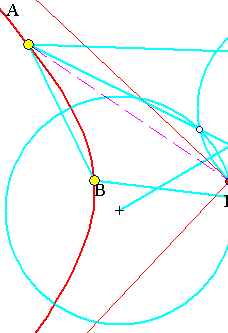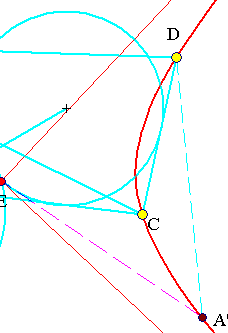##Rectangular Hyperbola through four points

Problem: Construct the rectangular hyperbola passing through four non-orthocentric points {A,B,C,D}.Orthocentric points are four points each tripple of which has the fourth point as orthocenter. Since a rectangular hyperbola circumscribing a triangle automatically passes through its orthocenter, all rectangular hyperbolas passing through three of the four points pass also through the fourth. Thus, four orthocentric points admit an infinite number of rectangular hyperbolas passing through them. The various hyperbolas are parameterized by the location of the center of the hyperbola on the Euler circle of one triangle formed by three of them (all such triangles have the same Euler circle). The corresponding picture for this case is discussed in RectHypeCircumscribed.html .
Four non orthocentric points (A, B, C, D) define four triangles having vertices three of them, in all possible combinations. The Euler circles of the resulting four triangles intersect at a common point E, shown above only for two out of the four circles (see EulerCirclesFour.html ). Necessarily E will be the center of the hyperbola, a fifth point will be the symmetric A' of A w.r. to E. The hyperbola can be constructed either by the method described in the above reference or through the tool constructing a conic passing through five points (A, B, C, D, A').